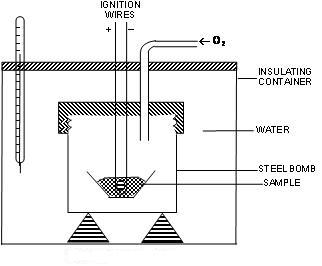Click to Chat

1800-1023-196

+91-120-4616500

CART 0

• 0

MY CART (5)

Use Coupon: CART20 and get 20% off on all online Study Material

ITEM
DETAILS
MRP
DISCOUNT
FINAL PRICE
Total Price: Rs.

There are no items in this cart.
Continue Shopping• Complete Physics Course - Class 11
• OFFERED PRICE: Rs. 2,968
• View Details

```BOMB CALORIMETER

The bomb calorimeter used for determining change in internal energy at constant volume if reaction for the combustion is known than enthalpy of combustion can be estimated by using formula: ΔH = ΔE + ΔnRT.Bomb Calorimeter

This apparatus was devised by Berthelot (1881) to measure the heat of combustion of organic compounds. A modified form of the apparatus shown in Figure consists of a sealed combustion chamber, called a bomb, containing a weighed quantity of the substance in a dish alongwith oxygen under about 20 atm pressure. The bomb is lowered in water contained in an insulated copper vessel. This vessel is provided with a stirrer and a thermometer reading up to 1/100th of a degree. It is also surrounded by an outer jacket to ensure complete insulation from the atmosphere. The temperature of water is noted before the substance is ignited by an electric current. After combustion, the rise in temperature of the system is noted on the thermometer and heat of combustion can be calculated from the heat gained by water and the calorimeter. By knowing the heat capacity of calorimeter and also the rise in temperature, the heat of combustion can be calculated by using the expression

Heat exchange = Z × ΔT

ΔT– rise in temp

Heat changes at constant volumes are expressed in ΔE and Heat changes at constant pressure are expressed in ΔH. Also, ΔH = ΔE + ΔnRT

Δn = Moles of gaseous product– Moles of gaseous reactant.

Sample problems. A sample of 0.16 g CH4 was subjected to combustion at 27°C in a bomb calorimeter. The temperature of the calorimeter system (including water) was found to rise by 0.5°C. Calculate the heart of combustion of methane at
(i) constant volume and (ii) constant pressure. The thermal capacity of calorimeter system is 17.7 KJ K-1 & R = 8.314 JK-1mol-1.

Solution: Heat of combustion at constant volume, ΔE = Heat capacity of calorimeter system× rise in temperature × Mol.mass of compound / mass of compound

= 17.7 × 0.5 × 16/0.16 = 885

ΔE = -885 kJ mol-1

CH4(g) + 2O2(g) ——> CO2 (g) + 2H2O(l)

Δn = 1 – 3 = -2, T = 300 K, R = 8.314 × 10-3 kJ K-1mol-1

ΔH = ΔE + ΔnRT

= –885 + (–2) × 8.314 × 10–3 kJk–1 mol–1

ΔH = ΔE + ΔnRT

= –885 + (–2) × 8.314 × 10–3 × 300

To read more, Buy study materials of Thermodynamics comprising study notes, revision notes, video lectures, previous year solved questions etc. Also browse for more study materials on Chemistry here.
```### Course Features

• 101 Video Lectures
• Revision Notes
• Previous Year Papers
• Mind Map
• Study Planner
• NCERT Solutions
• Discussion Forum
• Test paper with Video Solution